# uavCoverageSpace

2D coverage area for coverage planner

Since R2023a

## Description

The `uavCoverageSpace` object represents the coverage space as a set of convex polygons to use with the `uavCoveragePlanner` to perform coverage planning. Given a coverage area, the polygons form its configuration space.

## Creation

### Syntax

``space = uavCoverageSpace``
``space = uavCoverageSpace(Polygons=polygonVertices)``
``space = uavCoverageSpace(___,Name=Value)``

### Description

````space = uavCoverageSpace` creates an empty coverage space with default properties.```
````space = uavCoverageSpace(Polygons=polygonVertices)` creates a coverage space defined by convex one or more convex polygons as vertices `polygonVertices`, and sets the `Polygons` property.```

example

````space = uavCoverageSpace(___,Name=Value)` sets properties using one or more name-value arguments. For example, `uavCoverageSpace(Overlap=0.5)` sets the `Overlap` property to `0.5`.```

### Input Arguments

expand all

Convex polygons to survey, specified as an M-by-2 matrix or an N-element cell array of M-by-2 matrices. N is the total number of polygons, and M is the total number of vertices that define the polygon.

Concave polygons must be decomposed into convex polygons using the `coverageDecomposition` function.

The format of the vertices depends on the `UseLocalCoordinates` property:

• `UseLocalCoordinates=true` — Format is local xy-coordinates in the form [x y], in meters. `UseLocalCoordinates` is true by default.

• `UseLocalCoordinates=false` — Format is geodetic coordinates in the form [latitude longitude]. latitude and longitude are in degrees.

Example: `{[0 0; 0 1; 1 1; 1 0],[1 1; 2 2; 3 1]}`

Data Types: `single` | `double`

## Properties

expand all

Polygons to survey, specified as a N-element row vector of structures. N is the total number of polygons specified in the `polygons` argument. Each structure contains the `Vertices` and `SweepAngle`:

• `Vertices` — Vertices that define the polygon, specified as an M-by-2 matrix. M is the total number of vertices.

The format of the vertices depends on the `UseLocalCoordinates` property:

• `UseLocalCoordinates=true` — Format is local xy-coordinates in the form [x y], in meters. `UseLocalCoordinates` is true by default.

• `UseLocalCoordinates=false` — Format is geodetic coordinates in the form [latitude longitude]. latitude and longitude are in degrees.

• `SweepAngle` — Angle at which the UAV sweeps the polygon, specified as numeric scalar, in degrees. This value is NaN by default until you specify it or until the solver algorithm determines it when you plan the path with the `uavCoveragePlanner` object.

Overlap between sensor footprints in a single row, specified as a nonnegative numeric scalar in the range [0, 1). A value of `0` specifies that the sensor footprints have no overlap, and a value of `1` indicates that the sensor footprints fully overlap.

Data Types: `single` | `double`

Overlap between sensor footprints of two rows, specified as a nonnegative numeric scalar in the range [0, 1]. A value of `0` specifies that the sensor footprints have no sidelap, and a value of `1` specifies that the sensor footprints fully sidelap.

Data Types: `single` | `double`

Width of sensor footprint, specified as a positive numeric scalar, in meters. This measurement is along the sweep line direction.

Data Types: `single` | `double`

Length of sensor footprint, specified as a positive numeric scalar, in meters. This measurement is perpendicular to the sweep line direction.

Data Types: `single` | `double`

Flight altitude of UAV, specified as a nonnegative numeric scalar, in meters.

When `UseLocalCoordinates` is `true` or `1`, the reference height is with respect to the local reference frame. When `UseLocalCoordinates` is `false` or `0`, the coverage space assumes a flat-Earth approximation and the reference height is with respect to the ground at absolute zero meters.

Data Types: `single` | `double`

Use local coordinate or geodetic coordinate format, specified as `1` (`true`) for local coordinate format or `0` (`false`) for geodetic coordinate format.

• When `UseLocalCoordinates` is `true` or `1`:

• Vertices of polygons in the `uavCoverageSpace` are specified as xy-coordinates in the form [x y], in meters.

• `takeoff` and `landing` positions are specified as xyz-coordinates in the form [x y z], in meters.

• Planned waypoints are returned as xyz-coordinates in the form [x y z], in meters.

• When `UseLocalCoordinates` is `false` or `0`:

• Vertices of polygons in the `uavCoverageSpace` are specified as geodetic coordinates in the form [latitude longitude]. latitude and longitude are in degrees.

• `takeoff` and `landing` positions are specified as geodetic coordinates in the form [latitude longitude altitude]. latitude and longitude are in degrees, and altitude is in meters.

• Planned waypoints are returned as geodetic coordinates in the form [latitude longitude altitude]. latitude and longitude are in degrees, and altitude is in meters.

Georeference to compute and export to geodetic waypoint coordinates, specified as an three-element row vector of the form [latitude longitude altitude]. latitude and longitude are in degrees, and altitude is in meters.

Data Types: `double`

## Object Functions

 `setCoveragePattern` Set sweep angle for polygon in coverage area `show` Visualize 2D coverage space

## Examples

collapse all

This example shows how to plan a coverage path that surveys the parking lots of the MathWorks Lakeside campus.

Get the geodetic coordinates for the MathWorks Lakeside campus. Then create the limits for our map.

```mwLS = [42.3013 -71.375 0]; latlim = [mwLS(1)-0.003 mwLS(1)+0.003]; lonlim = [mwLS(2)-0.003 mwLS(2)+0.003];```

Create a figure containing the map with the longitude and latitude limits.

```fig = figure; g = geoaxes(fig,Basemap="satellite"); geolimits(latlim,lonlim)```

Get the outline of the first parking lot in longitude and latitude coordinates. Then create the polygon by concatenating them.

```pl1lat = [42.3028 42.30325 42.3027 42.3017 42.3019]'; pl1lon = [-71.37527 -71.37442 -71.3736 -71.37378 -71.375234]'; pl1Poly = [pl1lat pl1lon];```

Repeat the process for the second parking lot.

```pl2lat = [42.30035 42.2999 42.2996 42.2999]'; pl2lon = [-71.3762 -71.3734 -71.37376 -71.37589]'; pl2poly = [pl2lat pl2lon];```

Create the coverage space with both of those polygons, set the coverage space to use geodetic coordinates, and set the reference location to the MathWorks Lakeside campus location.

`cs = uavCoverageSpace(Polygons={pl1Poly,pl2poly},UseLocalCoordinates=false,ReferenceLocation=mwLS);`

Set the height at which to fly the UAV to 25 meters, and the sensor footprint width to 20 meters. Then show the coverage space on the map.

```ReferenceHeight = 25; cs.UnitWidth = 20; show(cs,Parent=g);```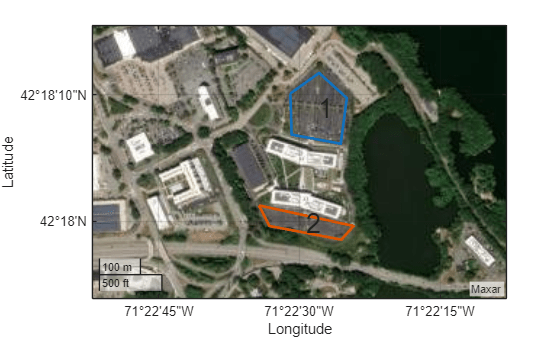Set the sweep angle for polygons 1 and 2 to `85` and `5` degrees, respectively, to have paths that are parallel to the roads in the parking lots. Then create the coverage planner for that coverage space with the exhaustive solver algorithm.

```setCoveragePattern(cs,1,SweepAngle=85) setCoveragePattern(cs,2,SweepAngle=5) cp = uavCoveragePlanner(cs,Solver="Exhaustive");```

Set the takeoff position to a location in the courtyard, then plan the coverage path.

```takeoff = [42.30089 -71.3752, 0]; [wp,soln] = plan(cp,takeoff); hold on geoplot(wp(:,1),wp(:,2),LineWidth=1.5); geoplot(takeoff(1),takeoff(2),MarkerSize=25,Marker=".") legend("","","Path","Takeoff/Landing") hold off```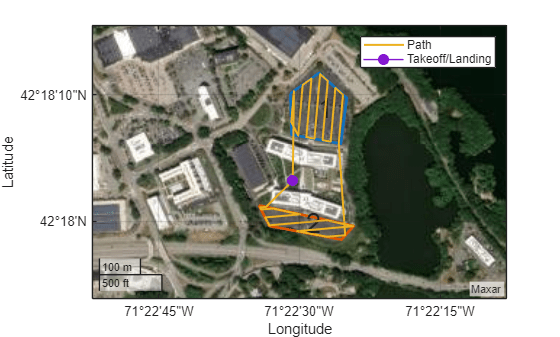This example shows how to plan a coverage path for a region in local coordinates and compares the results of using the exhaustive solver with the results of using the minimum traversal solver.

Define the vertices for a coverage space.

`area = [5 8.75; 5 27.5; 17.5 22.5; 25 31.25; 35 31.25; 30 20; 15 6.25];`

Because vertices define a concave polygon and the coverage planner requires convex polygons, decompose the polygon into convex polygons. Then create a coverage space with the polygons from decomposition.

```polygons = coverageDecomposition(area); cs = uavCoverageSpace(Polygons=polygons);```

Define the takeoff and landing positions at `[0 0 0]` and `[32.25 37.25 0]`, respectively. Then show the coverage space and plot the takeoff and landing positions.

```takeoff = [0 0 0]; landing = [32.25 37.25 0]; show(cs); exampleHelperPlotTakeoffLandingLegend(takeoff,landing)```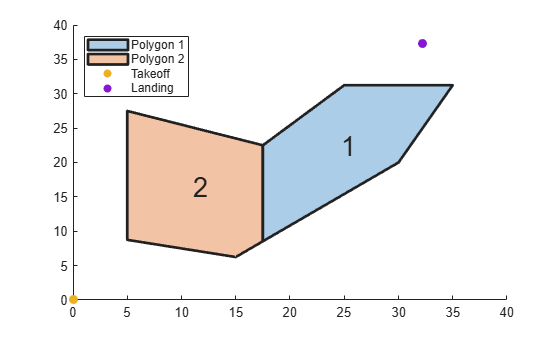Create a coverage planner with the exhaustive solver algorithm and another coverage planner with a minimum traversal solver algorithm. Because `Polygon 2` is closer to the takeoff position, set the visiting sequence of the solver parameters such that we traverse `Polygon 2` first.

```cpeExh = uavCoveragePlanner(cs,Solver="Exhaustive"); cpMin = uavCoveragePlanner(cs,Solver="MinTraversal"); cpeExh.SolverParameters.VisitingSequence = [2 1]; cpMin.SolverParameters.VisitingSequence = [2 1];```

Plan with both solver algorithms using the same takeoff and landing positions.

```[wptsExh,solnExh] = plan(cpeExh,takeoff,landing); [wptsMin,solnMin] = plan(cpMin,takeoff,landing);```

Show the planned path for both the exhaustive and the minimum traversal algorithms.

```figure show(cs); title("Exhaustive Solver Algorithm") exampleHelperPlotTakeoffLandingLegend(takeoff,landing,wptsExh)```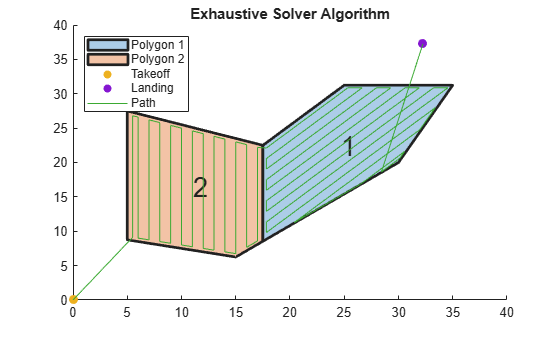```figure show(cs); title("Minimum Traversal Solver Algorithm") exampleHelperPlotTakeoffLandingLegend(takeoff,landing,wptsMin)```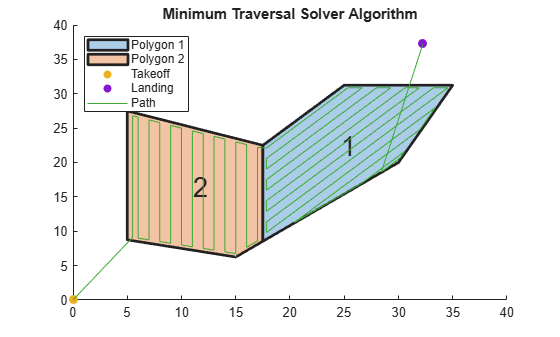Export the waypoints from the minimum traversal solver to a `.waypoints` file with the reference frame set to north-east-down.

`exportWaypointsPlan(cpMin,solnMin,"coveragepath.waypoints",ReferenceFrame="NED")`

## Version History

Introduced in R2023a

expand all